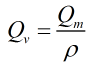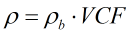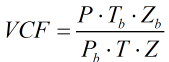# C261 – Gas Flowrate Conversion from Qm

## Description

This converts a gas mass flow rate to observed, standard or normal volume flow rate as well as energy flow rate.

Kelton calculation reference C261

## Options

### Calculate

• Volume flow rate
• Standard volume flow rate
• Normal volume flow rate
• Energy flow rate

This allows selection of what type of flow rate mass flow rate is to be converted to.

### Calorific Value

• Volume based
• Mass based

This option allows the user to enter either a volume or mass based calorific value when calculating energy flow rate.

### Method

• Density
• Volume correction factor

This option allows the user to select whether to calculate a volumetric type flow rate using the equivalent gas density or by using a volume correction factor.

### Volume Correction Factor

• User entered
• Calculated

This option allows the user to enter a volume correction factor or have it calculated using PTZ inputs.

### Standard Density

• User entered
• Input as relative density

This option allows the user to select whether to enter standard density or enter a relative density, which will then be used to calculate standard density.

## Calculation

### Volume Flow Rate

The volume flow rate is calculated by:If the density is calculated it is done by:If the volume correction factor is calculated it is done by:Where P = Pressure at line conditions Pb = Pressure at base conditions T = Temperature at line conditions Tb = Temperature at base conditions Z = Compressibility at line conditions Zb = Compressibility at base conditions ρ = Density at line conditions ρb = Density at base conditions Qm = Mass flow rate

### Energy Flow Rate

The energy flow rate is calculated by:

If volumetric calorific value is applied:If mass based calorific value is applied:Back to FLOCALC Calculations